# Problems of the area of a square

#### Number of problems found: 343

• ABS trianglePoint S is the center of the square ABCD. The ABS triangle has a content of 9 cm2. What is the circumference of the square in cm?
• Squares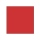From the square with the integer side, cut out the square with the integer side so that the residual area is 100. What is the longest possible side of the larger square?
• The widthThe width of a rectangular garden is 4 m less than the length. If the area of a rectangular garden is 96 square meters, what is the dimension of the garden?
• Semicircle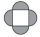The ornament consists of one square and four dark semicircles. The area of the square is 4 cm ^ 2. Find the area of one dark semicircle and round the result to hundreds.
• AlexandraAlexandra made a rectangular quilt the measured 3 1/4. 2 3/4 feet in width. What is the area of the quilt in square feet? Write an equation to solve.
• ParallogramThe Parallelogram base is 24 cm and high 10 cm. How many are such tiles required to cover a floor of area 1080m2?
• A trapezoid 3A trapezoid ABCD has the bases length of a = 120 mm, c = 86 mm and the area A = 2,575 mm2. Find the height of the trapezoid.
• Perimeter to area 2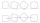Find the area of a square whose perimeter is 100 cm with solve.
• The area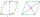The area of a rhombus is 143 m2. If the longer diagonal is 26 m, find the shorter diagonal in inches named d1.
• Cups of paintI have a board that is 2 meters wide and 3 meters tall. If one cup of paint cover 1,000 square centimeters. How many cups of paints does I need?
• Little 2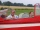Little fields farm lies on a rectangular plot of land that measures 2 2/4 miles in length and 2 2/3 miles in width. What is it's area in square miles?
• Find the 28Find the area of a square with a side length of 4/5 yards.
• Flowerbeds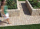There are three square flower beds on the square grassy plot. What percentage is the grassed part of the land, when one side of the land is 35 m and the side of the flowerbed is 10 m?
• Louis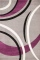Louis wants to carpet the rectangular floor of his basement. The basement has an area of 5,120 square feet. The width of the basement is 4/5 its length. What is the length of Louis's basement?
• AmeekaAmeeka is in charge of designing a school pennant for spirit week. She wants the base to be 3 1/2 feet and the height to be 6 1/2 feet. She has 20 square feet of paper available. Does she have enough paper?
• Paper cuttingSusanna is cutting construction paper into rectangles for a project. She needs to cut one rectangle that is 10 inches × 14 1/2 inches. She needs to cut another rectangle that is 10 1/3 inches by 10 1/2 inches. How many total square inches of construction
• Goat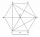The fenced flower bed has the shape of a regular hexagon. The tops are formed by fence posts. The fence around the flowerbed measures 60 m. A goat is tied to one of the pillars from the outside and grazes on the surrounding meadow (the goat should not ent
• Mr DumaMr Duma recently inherited a rectangular plot, part of the estate left by his late father. The plot with the following dimensions: Length=2x+1;Width=x-1. Determine the formula, in terms of x, that best describes the area of the rectangular plot. He has pl
• Living roomMarina's new living room is a rectangle with a length of 8 meters and a width of 5 meters. She wants to buy a carpet to cover half of the living room and each square meter of the carpet has a cost of \$4. How much will be the total cost for Marina?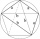The quadrilateral ABCD is composed of two right triangles ABD and BCD. For side lengths: |AD| = 3cm, | BC | = 12cm, | BD | = 5cm. How many square centimeters (area) does the quadrilateral ABCD have? The angles DAB and DBC are right.